Question

# Figure shows three systems. System I is a control system proportional. System II is a position control system with PD control action. System III is a speed feedback position control system. Compare the unit step, unit impulse, and unit ramp responses o

Figure shows three systems. System I is a control system

proportional. System II is a position control system with PD control action.

System III is a speed feedback position control system.

Compare the unit step, unit impulse, and unit ramp responses of the three

systems. (Analytically and using Matlab's Simulink, to compare the results)

system is better with respect to speed of response and maximum overshoot in the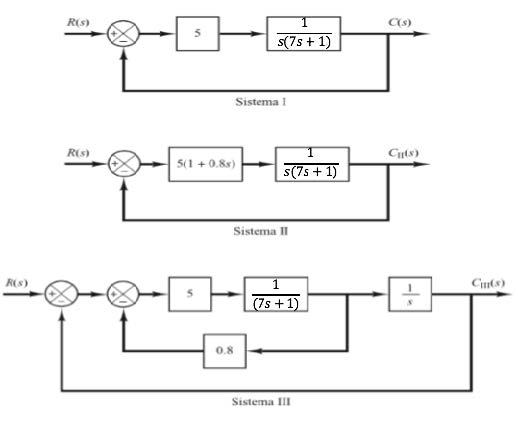We need at least 10 more requests to produce the answer.

0 / 10 have requested this problem solution

The more requests, the faster the answer.

All students who have requested the answer will be notified once they are available.

#### Earn Coins

Coins can be redeemed for fabulous gifts.

Similar Homework Help Questions
• ### ControlFigure shows three systems. System I is a control systemproportional. System II is a position control system with PD control action.System III is a speed feedback position control system.Compare the unit step, unit impulse, and unit ramp responses of the threesystems. (Analytically and using Matlab's Simulink, to compare the results)system is better with respect to speed of response and maximum overshoot in thestep answer?

• ### 6, Fig. 4 shows three systems. System 1 is a positional servo system. System Π is a positional servo system with PD con...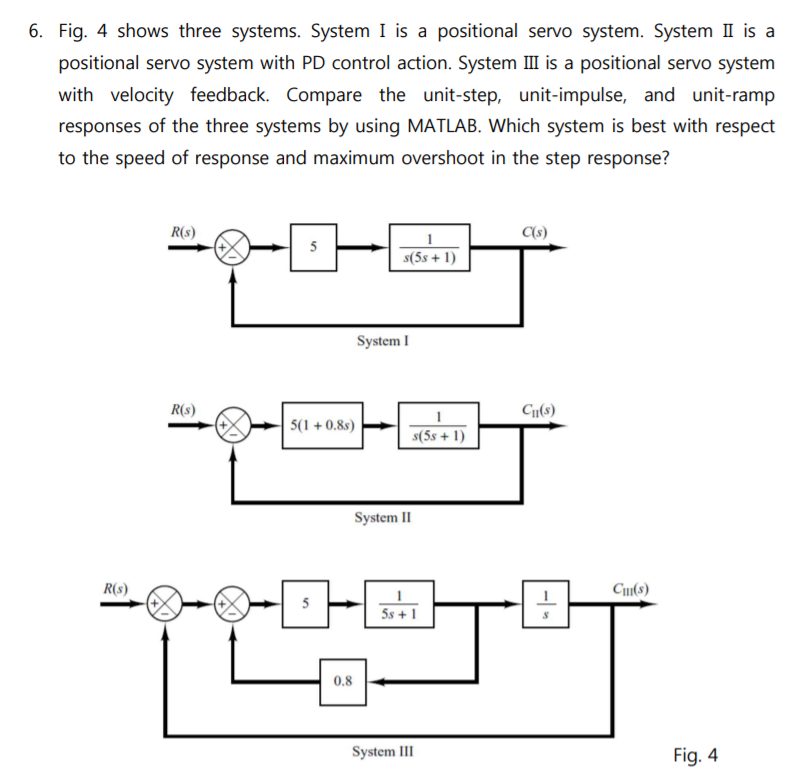6, Fig. 4 shows three systems. System 1 is a positional servo system. System Π is a positional servo system with PD control action. System III is a positional servo system with velocity feedback. Compare the unit-step, unit-impulse, and unit-ramp responses of the three systems by using MATLAB. Which system is best with respect to the speed of response and maximum overshoot in the step response? R(s) C(s) #5s + 1) System I R(s) CI(s S1 +0.8)6-D) System II R(s)...

• ### Compare unit step responses for the following three systems and see how “zero” affects transient response...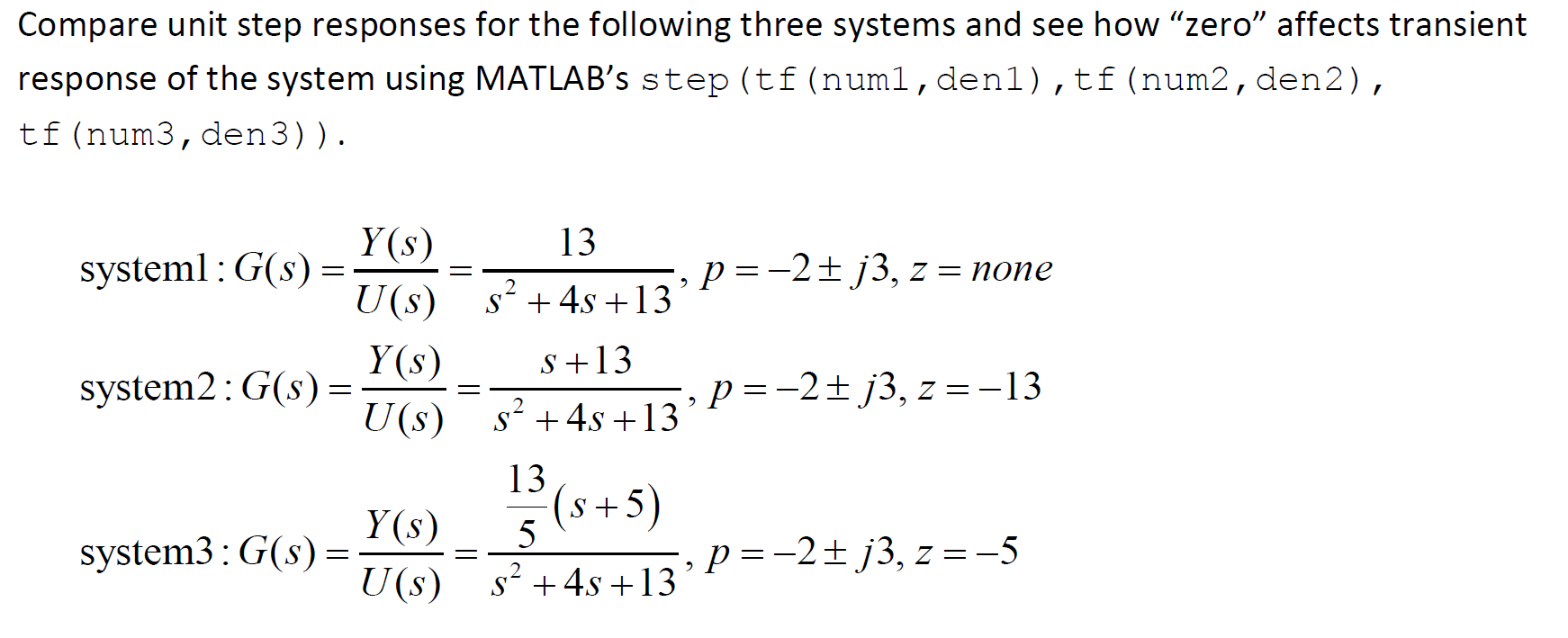Compare unit step responses for the following three systems and see how “zero” affects transient response of the system using MATLAB's step (tf (numl, den1),tf (num2, den2), tf (num3, den3)). 13 systeml: G(s)-Y(S) U(s) Y(S) system2:G(S) = U(s) -, p =-2+ j3, z = none \$? +45 +13° S+13 5, p =-2j3, z=-13 s? +45 +13' (5+5) system3: G(S)= (s) s? +45 +13' 5, P =-2 + j3, z =-5

• ### ' 1. Review Question a) Name three applications for feedback control systems. b) Functionally, ho...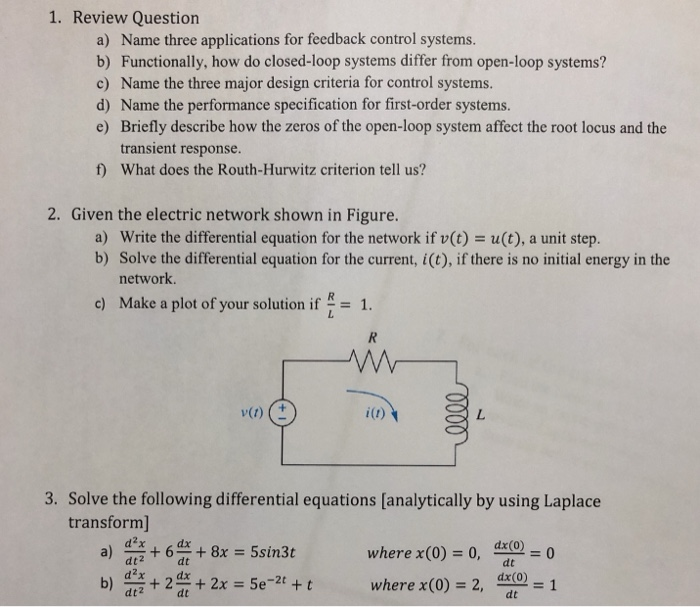' 1. Review Question a) Name three applications for feedback control systems. b) Functionally, how do closed-loop systems differ from open-loop systems? c) Name the three major design criteria for control systems. d) Name the performance specification for first-order systems. e) Briefly describe how the zeros of the open-loop system affect the root locus and the transient response. What does the Routh-Hurwitz criterion tell us? f) 2. Given the electric network shown in Figure. a) Write the differential equation for...

• ### Question three The figure below shows a unit step response of a second order system. From...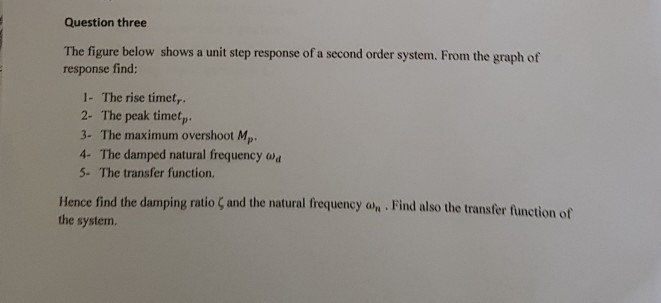Question three The figure below shows a unit step response of a second order system. From the graph of response find: 1- The rise timet, 2- The peak timet, 3- The maximum overshoot Mp 4- The damped natural frequency w 5. The transfer function. Hence find the damping ratio ζ and the natural frequency ah-Find also the transfer function of the system. r 4 02 15 25 35 45 Question Four For the control system shown in the figure below,...

• ### Q.4 A position control system is shown in Figure Q4. Assume that K(s) = K, the plant 50 s(0.2s +1) transfer function is given by G(s) s02s y(t) r(t) Figure Q4: Feedback control system. (a) Design a l...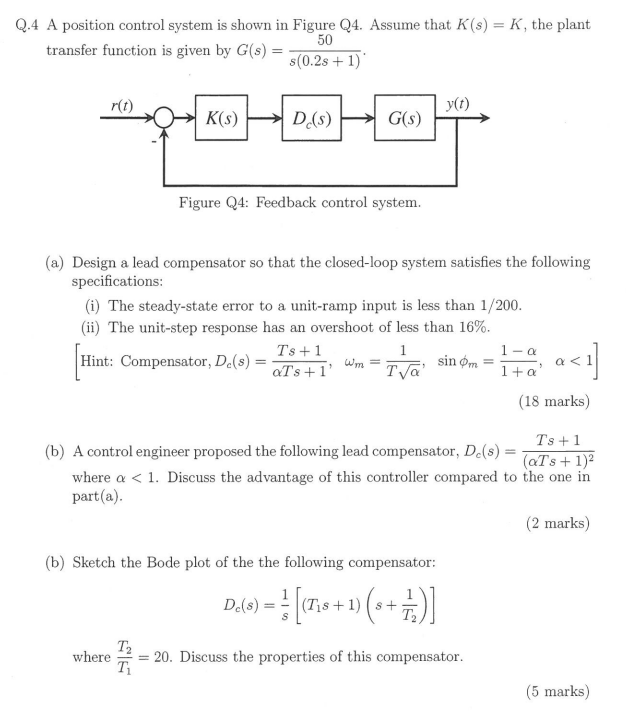Q.4 A position control system is shown in Figure Q4. Assume that K(s) = K, the plant 50 s(0.2s +1) transfer function is given by G(s) s02s y(t) r(t) Figure Q4: Feedback control system. (a) Design a lead compensator so that the closed-loop system satisfies the following specifications (i) The steady-state error to a unit-ramp input is less than 1/200 (ii) The unit-step response has an overshoot of less than 16% Ts +1 Hint: Compensator, Dc(s)=aTs+ 1, wm-T (18 marks)...

• ### PLEASE DO IN MATLAB Problem 8 (PID feedback control). This problem is about Proportional-Integral-Derivative feedback control...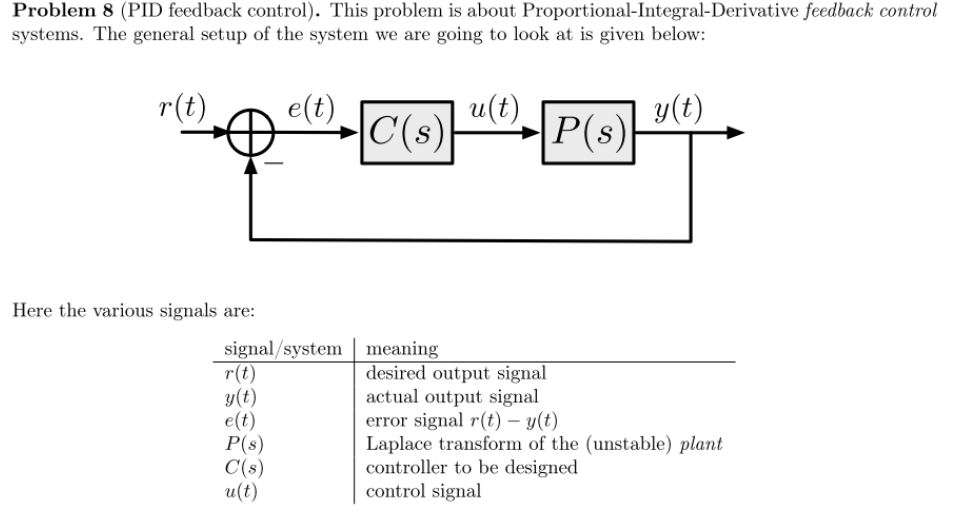PLEASE DO IN MATLAB Problem 8 (PID feedback control). This problem is about Proportional-Integral-Derivative feedback control systems. The general setup of the system we are going to look at is given below: e(t) u(t) |C(s) y(t) P(s) r(t) Here the various signals are: signal/system r(t) y(t) e(t) P(s) C(s) и(t) meaning desired output signal actual output signal error signal r(t) y(t) Laplace transform of the (unstable) plant controller to be designed control signal Our goal is to design a controller...

• ### PROBLEM 4 Suppose that a system is shown in Figure 4. There are three controllers that might be incorporated into this system. 1. Ge (s)-K (proportional (P) controller) 2. GS)K/s (integral (I) contro...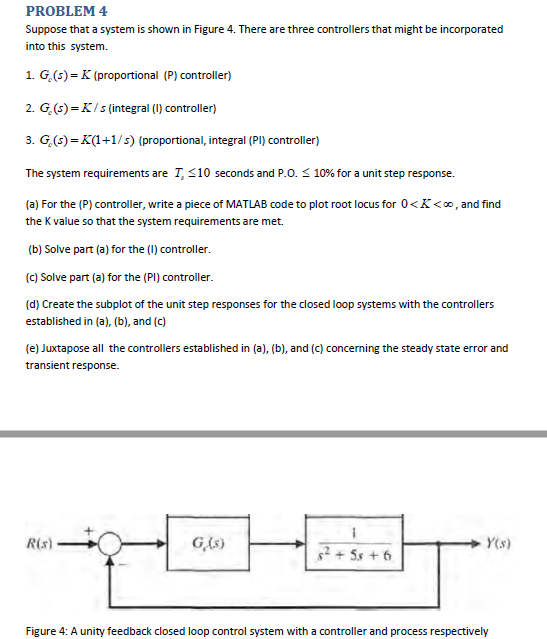PROBLEM 4 Suppose that a system is shown in Figure 4. There are three controllers that might be incorporated into this system. 1. Ge (s)-K (proportional (P) controller) 2. GS)K/s (integral (I) controller) 3. G (s)K(1+1/s) (proportional, integral (PI) controller) The system requirements are T, < 10 seconds and P0 10% for a unit step response. (a) For the (P) controller, write a piece of MATLAB code to plot root locus for 0<K<,and find the K value so that the...